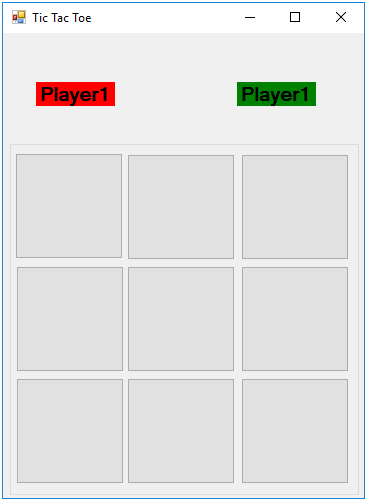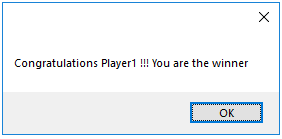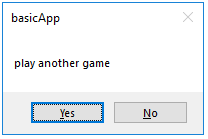# Tic Tac Toe Mini VB.NET Project

Tic Tac Toe is a game played by two players on a grid of 3X3 squares. The challenge is to place three consecutive Os or Xs by one player in a row, column or diagonal. The player who does so is the winner. The game ends in a draw if all the squares are filled with Os or Xs but not in a row, column or diagonal. We will create the Tic Tac Toe Mini VB.NET Project to let you understand the concept of event handling and coding in VB.NET

The design interface of this game is created with 9 buttons arranged in 3 rows and 3 columns. When a button is clicked by a player its colour changes according to the colour assigned to players in the labels Player1 and Player2.Controls and their names

Three labels

• Label1- to store the array storing which player clicked which button from index 1 to 9. Its Visible property is set to false
• Label2 – Text property Player1 and backcolor property Red
• Label3 – Text property Player2 and backcolor property Green

Nine Buttons– Button1 to Button9

## Working of Tic Tac Toe Mini VB.NET Project

When the game is executed the interface is presented to the players who can take turn to click one out of nine buttons. As soon as a button is clicked the button changes its color. When one player succeeds in drawing three consecutive buttons (row/column/diagonal) with his assigned color he is declared a winner. A message box is displayed with the player who wins.After finishing a game the players are asked if they wish to play again. On clicking yes button the buttons are set to the basic color and the players can start again.## Code Implementation

Variable declaration for Tic Tac Toe Mini VB.NET Project

```    Dim player1, player2 As Boolean ‘ indicate which player has played his turn
Dim bc() As Integer ‘ for storing which button is clicked by which players(1/2)
Dim i As Integer ‘ counter for for loop
Dim winn As Integer ‘ storing the player number of winner
Dim tot As Integer
Dim fin As Boolean```

Form load event to initialize the variables

``` Private Sub tictactoe_Load(sender As Object, e As EventArgs) Handles Me.Load
Dim i As Integer
ReDim bc(8)
For i = 0 To 8
bc(i) = 0
Next
i = 0
tot = 0
player1 = True
player2 = False
winn = 0
fin = True
btnRefresh.Focus()
End Sub```

Refresh Button code

``` Private Sub btnRefresh_Click(sender As Object, e As EventArgs) Handles btnRefresh.Click
tttReset()
End Sub```

Button1 to button 9 click are handled by one click event

``` Private Sub Button1_Click(sender As Object, e As EventArgs) Handles Button1.Click, Button2.Click, Button3.Click, Button4.Click, Button5.Click, Button6.Click, Button7.Click, Button8.Click, Button9.Click
Dim sname As String
Dim res
sname = CStr(sender.name)
i = Mid(sname, Len(sname), 1) - 1

If player1 Then
sender.BackColor = Color.Red
player1 = False
player2 = True
bc(i) = 1
Else
sender.BackColor = Color.Green
player1 = True
player2 = False
bc(i) = 2
End If
Label1.Text = ""
For i = 0 To 8
Label1.Text = Label1.Text + CStr(bc(i))
Next
'checking all rows

If Mid(Label1.Text, 1, 3) = "111" Then
winn = 1
End If
If Mid(Label1.Text, 1, 3) = "222" Then
winn = 2
End If
If Mid(Label1.Text, 7, 3) = "111" Then
winn = 1
End If
If Mid(Label1.Text, 7, 3) = "222" Then
winn = 2
End If
If Mid(Label1.Text, 4, 3) = "111" Then
winn = 1
End If
If Mid(Label1.Text, 4, 3) = "222" Then
winn = 2
End If

'checking all columns
If CStr(bc(0)) + CStr(bc(3)) + CStr(bc(6)) = "111" Then
winn = 1
End If
If CStr(bc(0)) + CStr(bc(3)) + CStr(bc(6)) = "222" Then
winn = 2
End If
If CStr(bc(1)) + CStr(bc(4)) + CStr(bc(7)) = "111" Then
winn = 1
End If
If CStr(bc(1)) + CStr(bc(4)) + CStr(bc(7)) = "222" Then
winn = 2
End If
If CStr(bc(2)) + CStr(bc(5)) + CStr(bc(8)) = "111" Then
winn = 1
End If
If CStr(bc(2)) + CStr(bc(5)) + CStr(bc(8)) = "222" Then
winn = 2
End If

'checking all diagonal positions
If CStr(bc(0)) + CStr(bc(4)) + CStr(bc(8)) = "111" Then
winn = 1
End If
If CStr(bc(0)) + CStr(bc(4)) + CStr(bc(8)) = "222" Then
winn = 2
End If
If CStr(bc(2)) + CStr(bc(4)) + CStr(bc(6)) = "111" Then
winn = 1
End If
If CStr(bc(2)) + CStr(bc(4)) + CStr(bc(6)) = "222" Then
winn = 2
End If

'printing winners name and message
If winn = 1 Or winn = 2 Then
MessageBox.Show("Congratulations Player" & CStr(winn) & " !!! You are the winner")
res = MsgBox("play another game", vbYesNo)

If res = vbYes Then
tttReset()
Else
Me.Close()
End If
Else

For i = 0 To 8
If bc(i) <> 0 Then
fin = True
Else
fin = False
End If
Next
If fin = True Then
MessageBox.Show("Its a Draw")

res = MsgBox("play another game", vbYesNo, Me.Text)

If res = vbYes Then
tttReset()
Else
Me.Close()
End If
End If
End If
End Sub```

User define procedure to refresh the game Tic Tac Toe Mini VB.NET Project

``` Private Sub tttReset()
Label1.Text = ""
winn = 0
tot = 0
fin = True
For i = 0 To 8
bc(i) = 0
Next
If winn = 2 Then
player2 = True
player1 = False
Else
player1 = True
player2 = False
End If
Button1.BackColor = DefaultBackColor
Button2.BackColor = DefaultBackColor
Button3.BackColor = DefaultBackColor
Button4.BackColor = DefaultBackColor
Button5.BackColor = DefaultBackColor
Button6.BackColor = DefaultBackColor
Button7.BackColor = DefaultBackColor
Button8.BackColor = DefaultBackColor
Button9.BackColor = DefaultBackColor
End Sub```